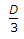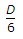# Civil Engineering - Estimating and Costing

## Why Civil Engineering Estimating and Costing?

In this section you can learn and practice Civil Engineering Questions based on "Estimating and Costing" and improve your skills in order to face the interview, competitive examination and various entrance test (CAT, GATE, GRE, MAT, Bank Exam, Railway Exam etc.) with full confidence.

## Where can I get Civil Engineering Estimating and Costing questions and answers with explanation?

IndiaBIX provides you lots of fully solved Civil Engineering (Estimating and Costing) questions and answers with Explanation. Solved examples with detailed answer description, explanation are given and it would be easy to understand. All students, freshers can download Civil Engineering Estimating and Costing quiz questions with answers as PDF files and eBooks.

## Where can I get Civil Engineering Estimating and Costing Interview Questions and Answers (objective type, multiple choice)?

Here you can find objective type Civil Engineering Estimating and Costing questions and answers for interview and entrance examination. Multiple choice and true or false type questions are also provided.

## How to solve Civil Engineering Estimating and Costing problems?

You can easily solve all kind of Civil Engineering questions based on Estimating and Costing by practicing the objective type exercises given below, also get shortcut methods to solve Civil Engineering Estimating and Costing problems.

### Exercise :: Estimating and Costing - Section 1

1.

Pick up the excavation where measurements are made in square metres for payment.

 A. Ordinary cuttings up to 1 m B. surface dressing up to 15 cm depths C. Surface excavation up to 30 cm depths D. Both (b) and (c) E. Both (a) and (b)

Explanation:

No answer description available for this question. Let us discuss.

2.

The expected out turn of 2.5 cm cement concrete floor per manson per day

 A. 2.5 sqm B. 5.0 sqm C. 7.5 sqm D. 10 sqm

Explanation:

No answer description available for this question. Let us discuss.

3.

A portion of an embankment having a uniform up-gradient 1 in 500 is circular with radius 1000 m of the centre line. It subtends 180° at the centre. If the height of the bank is 1 m at the lower end, and side slopes 2:1, the earth work involved.

 A. 26, 000 m3 B. 26, 500 m3 C. 27, 000 m3 D. 27, 500 m3

Explanation:

No answer description available for this question. Let us discuss.

4.

The correct prismoidal formula for volume is

 A. D [first area + last area + ∑ Even area + 2 ∑ odd areas] B.[first area + last area + 4 ∑ Even area + 2 ∑ odd areas] C.[first area + last area + 2 ∑ Even area + 4 ∑ odd areas] D.[first area + last area + 2 ∑ Even area + 4 ∑ odd areas].

Explanation:

No answer description available for this question. Let us discuss.

5.

The order of booking dimensions is